# Advanced Econometrics: Quantiles and Expectiles

Next Thursday, I will give the fourth lecture of the PhD course on advanced tools for econometrics, on quantile and expectile regressions. Slides are available online.

# Variance of the Average of a Sequence

In the case where  are i.i.d. random variables, then

Now, what if  are identically distributed, but no longer independent. What if we have an autoregressive process? Assume that

Then

can be written

Here, we will express the variance as a function of  and , but it is possible to use also , since, in the context of an ,

Now, since  we get

which can be simplified, since

i.e.

So, the variance of the mean can be writen as

Observe that if  is large enough,

This asymptotic relationship is well known actually. A simple way to get it is the following. One can can write

or equivalently

But actually, the first relationship is probably more interesting to get an asymptotic approximation,

In the context of an  process, this can be writen

Thus, we get the following well-known relationship

In the case where  is an i.i.d. sequence, i.e. , then we get the relationship mentioned initially. And in the case of a random walk… unfortunately, we cannot use that relationship. But observe that

i.e.

which can be written

If we compare the true value and the approximation, we get the following graph,

> V=function(phi,s2=1,n=100){
+ g0=s2/(1-phi^2)
+ if(phi<1){
+ if(phi==0){v1=g0/n}
+ if(phi>0){v1=g0/n^2*(n+2*((n-1)*
+ phi^(-1)-n+phi^(n-1))/(phi^(-1)-1)^2)}
+ v2=g0/n*(1+phi)/(1-phi)
+ }
+ if(phi==1){
+ v1=(2*n+1)*(n+1)*s2/(6*n)
+ v2=NA
+ }
+ return(c(v1,v2))}
>
> Vphi=function(phi) V(phi,1,100)
> x=seq(.01,1,by=.02)
> M=matrix(unlist(lapply(x,V)),nrow=2)
> plot(x,M[1,],type="l",col="red",log="y",
+ ylab="Variance of the average (log scale)",
+ xlab="Autoregressive coefficient")
> lines(x,M[2,],col="blue")# Tests on tail index for extremes

Since several students got the intuition that natural catastrophes might be non-insurable (underlying distributions with infinite mean), I will post some comments on testing procedures for extreme value models.

A natural idea is to use a likelihood ratio test (for composite hypotheses). Letdenote the parameter (of our parametric model, e.g. the tail index), and we would like to know whetheris smaller or larger than(where in the context of finite versus infinite mean). I.e. eitherbelongs to the setor to its complementary. Consider the maximum likelihood estimator, i.e.Letanddenote the constrained maximum likelihood estimators onandrespectively,Eitherand(on the left), orand(on the right)So likelihood ratiosare either equal toorIf we use the code mentioned in the post on profile likelihood, it is easy to derive that ratio. The following graph is the evolution of that ratio, based on a GPD assumption, for different thresholds,

> base1=read.table(
+ "http://freakonometrics.free.fr/danish-univariate.txt",
> library(evir)
> X=base1$Loss.in.DKM > U=seq(2,10,by=.2) > LR=P=ES=SES=rep(NA,length(U)) > for(j in 1:length(U)){ + u=U[j] + Y=X[X>u]-u + loglikelihood=function(xi,beta){ + sum(log(dgpd(Y,xi,mu=0,beta))) } + XIV=(1:300)/100;L=rep(NA,300) + for(i in 1:300){ + XI=XIV[i] + profilelikelihood=function(beta){ + -loglikelihood(XI,beta) } + L[i]=-optim(par=1,fn=profilelikelihood)$value }
+ plot(XIV,L,type="l")
+ PL=function(XI){
+ profilelikelihood=function(beta){
+ -loglikelihood(XI,beta) }
+ return(optim(par=1,fn=profilelikelihood)$value)} + (L0=(OPT=optimize(f=PL,interval=c(0,10)))$objective)
+ profilelikelihood=function(beta){
+ -loglikelihood(1,beta) }
+ (L1=optim(par=1,fn=profilelikelihood)$value) + LR[j]=L1-L0 + P[j]=1-pchisq(L1-L0,df=1) + G=gpd(X,u) + ES[j]=G$par.ests
+ SES[j]=G$par.ses + } > > plot(U,LR,type="b",ylim=range(c(0,LR))) > abline(h=qchisq(.95,1),lty=2)with on top the values of the ratio (the dotted line is the quantile of a chi-square distribution with one degree of freedom) and below the associated p-value > plot(U,P,type="b",ylim=range(c(0,P))) > abline(h=.05,lty=2)In order to compare, it is also possible to look at confidence interval for the tail index of the GPD fit, > plot(U,ES,type="b",ylim=c(0,1)) > lines(U,ES+1.96*SES,type="h",col="red") > abline(h=1,lty=2)To go further, see Falk (1995), Dietrich, de Haan & Hüsler (2002), Hüsler & Li (2006) with the following table, or Neves & Fraga Alves (2008). See also here or there (for the latex based version) for an old paper I wrote on that topic. # It is “simply” the average value For some obscure reasons, simple things are usually supposed to be simple. Recently, on the internet, I saw a lot of posts on the “average time in which you hold a stock“, and two rather different values are mentioned • Take any stock in the United States. The average time in which you hold a stock is – it’s gone up from 20 seconds to 22 seconds in the last year” (Michael Hudson on http://www.telegraph.co.uk/) or “The founder of Tradebot, in Kansas City, Mo., told students in 2008 that his firm typically held stocks for 11 seconds” (on http://www.nytimes.com/) among many others • Based on the NYSE index data, the mean duration of holding period by US investors was around 7 years in 1940. This stayed the same for the next 35 years. The average holding period had fallen to under 2 years by the time of the 1987 crash. By the turn of the century it had fallen to below one year. It was around 7 months by 2007” (on http://topforeignstocks.com/ see also the graph below) or “Two-thirds [of the managers of more than 800 institutional funds interviewed in a study] had higher turnover than they predicted […] Even though most are judged by performance over three-year horizons, their average holding period was about 17 months, and 19% of the managers held the typical stock for one year or less” (mentioned on http://online.wsj.com/) again among many others How comes that on the one hand, some people talk about less than 20 sec. for the “average time in which you hold a stock“, and on the other, around a year. How can we have such a difference ? We are talking about an average time here, not a rare event probability… To understand what might be wrong, consider the following case, with a market, and two stocks: one is kept over a year (52 weaks) while the other other is traded – and exchanged – every week (52 times per year). What is the “average time in which you hold a stock” ? Is it • 26.5 weeks ? the average time for the first stock is 52 weeks, while it is 1 for the second one, i.e. 53 over 2 • 1.96 weeks ? over a year the first stock has been traded once, while it was exchanged 52 times for the second one, i.e. 104 over 53 (total time over the total number of transactions) Obviously, there is a selection bias in that study (see here for an illustration of that concept, in French). In order to get a better understanding, consider the following simple model, with a large number of simulated stocks. At each transaction, they can be hold by 3 types of investors, • with probability 70%, hold – on average – for 20 sec. • with probability 20%, hold – on average – for 15 days • with probability 10%, hold – on average – for 10 years As claimed by Warren Buffett, “my favorite time frame for holding a stock is forever“, so it might not be absurd to consider investors who keep a stock for a long period of time. Assume further that the time frame for holding a stock is exponentially distributed (the rate depending on the kind of investor). Assume that those stocks are observed during a period of time of 20 years (which might sound reasonable). Several techniques can be used to estimate the “average time in which you hold a stock • The first one is to calculate the mean, per stock, of the holding time, and to consider the average over all the stocks. Maybe it would be a good idea to exclude the last observation (since data were censored), • The second one is to divide the (total) period of time by the (total) number of investors that hold the stock during that time frame (or number of transactions) • A third idea might be to use the first method, but instead of removing the last one, to use an estimator of the mean based on Kaplan-Meier estimate • A fourth idea is to look at what happened at a specific date (say after 10 years), i.e. which investor had the stock, and how long he kept it. The code to generate that process is the following > set.seed(1) > invest=sample(size=ns,c("A","B","C"), + prob=c(.7,.2,.1),replace=TRUE) > lambda=(invest=="A")*20/(365*24*60*60)+ + (invest=="B")*15/365+ + (invest=="C")*10 > E=rexp(ns,rate=1/lambda) > T=cumsum(E) > T=T[T<20] > plot(c(T,50),0:length(T),type="s",xlim=c(0,20),col="blue") with the following trajectory for the number of investor that did hold that specific stock between time 0 and time 20.Then, the different techniques are the following, # method 1 > E1=diff(T) > m1=mean(E) > M1[s]=m1 for the first one (means of time length, per stock), # method 2 > if(length(T)>1){ + n2=length(T)-1 + d2=T[length(T)-l]-T + N2[s]=n2; D2[s]=d2 + } for the second one (time length and number of transactions), + # method 3 + T3=c(T,20) + C3=c(rep(0,length(T)-1),1) + km=survfit(Surv(diff(T3), 1-C3)~1) + m3=summary(km,rmean='individual')$table
+ M3[s]=m3

for the third one (based on a prediction of the expected mean, from Kaplan-Meier estimate) and

# method 4
> T0=c(0,T,20)
> m4=min(T0[T0>10])-max(T0[T0<10])
> M4[s]=m4

for the fourth one (based on what happened at time 10). Using monte carlo simulations, we get very different quantities, that can all be interpreted as the “average time in which you hold a stock”

> sum(D2,na.rm=TRUE)/sum(N2,na.rm=TRUE)
 0.3692335
> mean(M1,na.rm=TRUE)
 0.5469591
> mean(M3,na.rm=TRUE)
 1.702908
> mean(M4,na.rm=TRUE)
 12.40229

If we change to probabilities (and assume that high frequency investors are much more important than long-term ones), e.g.

> invest=sample(size=ns,c("A","B","C"),
+ prob=c(.9,.09,.01),replace=TRUE)

then the first two estimates are rather different. But not the last two.

> sum(D2,na.rm=TRUE)/sum(N2,na.rm=TRUE)
 0.04072227
> mean(M1,na.rm=TRUE)
 0.06393767
> mean(M3,na.rm=TRUE)
 0.2504322
> mean(M4,na.rm=TRUE)
 12.05508

So I have to confess that the “average time in which you hold a stock” can be almost anything from 10 sec. to 10 years, it clearly depends on the way the average is calculated. The second point is that if the proportion of high frequency trading is extremely high, I should not affect the last one (which is, from my point of view, the most interesting one, an might also be improved by here also integrate a censored variate). So I guess people should be careful when discussing such quantities… And if anyone is willing to share data on that topic, I’d be glad to look at them…

# “standardized” version of the maximum

For the first homework, there was a tricky question in problem 29, chapter 5. Here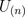is the maximum of n random variables i.i.d. uniformly distributed on the unit interval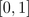. I gave a hint last week about the cumulative distribution function for the maximum, i.e.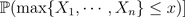is equal to the probability that all are smaller than,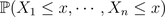Then, we use independent to obtain that this probability is a product, of equal quantities since all random variables are identically distributed, i.e.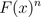Then, the exercise ask the followingi.e. find a standardized version of that the maximum so that the cumulated distribution of that standardized version has a (non degenerated) limiting value. A hint is given in the answers, at the end of the book,Actually, the question is not that simple (see here for the history of that question).
What I said during the course is that if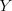is a random variable with finite variance, thenis a standardized (or normalized) version of, in the sense that it is centered, i.e.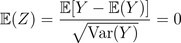and with a unit variance, i.e.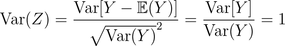This is the kind of standardization (or normalization) that is used in the central limit theorem i.e. it is interesting when we study the core of our distribution (i.e. the mean).
Here we focus on the maxima (not on the expected value). Note that here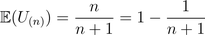while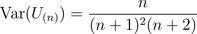(up to some typing mistakes). Thus, our previous standardization would be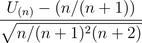that can be simplified as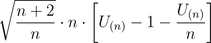Hence, that random variable can be approximated by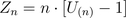since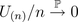as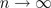. Here, it is then possible to get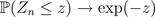since if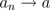, then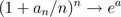(see the prof of the central limit theorem we got a few days ago).
But this is usually not the way we work with maxima. Actually, Fréchet, Fisher, Tippett, Gnedenko proved that the appropriate standardization to work with maxima is to consider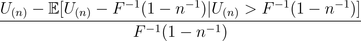where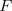is the cumulative distribution of the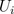‘s (the random variables used to build up the maximum). This work since the‘s have a finite support, i.e. theare bounded, with an upper limit (here 1).
Note that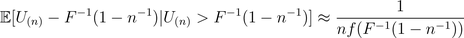assuming that the density associated withexists. Hence, here the standardization becomes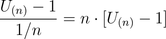which is exactly the one that John Rice is suggesting… And the proper motivation comes from extreme value theory, but it is a bit far away from what we shall see in that course…